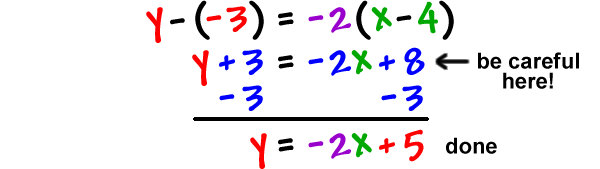If we have a point,, and a slope, m, here's the formula we use to find the equation of a line:It's called the point-slope formula
(Duh!)

You are going to use this a LOT!

Luckily, it's pretty easy -- let's just do one:

Let's find the equation of the line that passes through the point
( 4 , -3 ) with a slope of -2:Just stick the stuff in a clean it up!TRY IT:

Find the equation of the line that passes through the point ( -2 , 6 ) with a slope of 3.